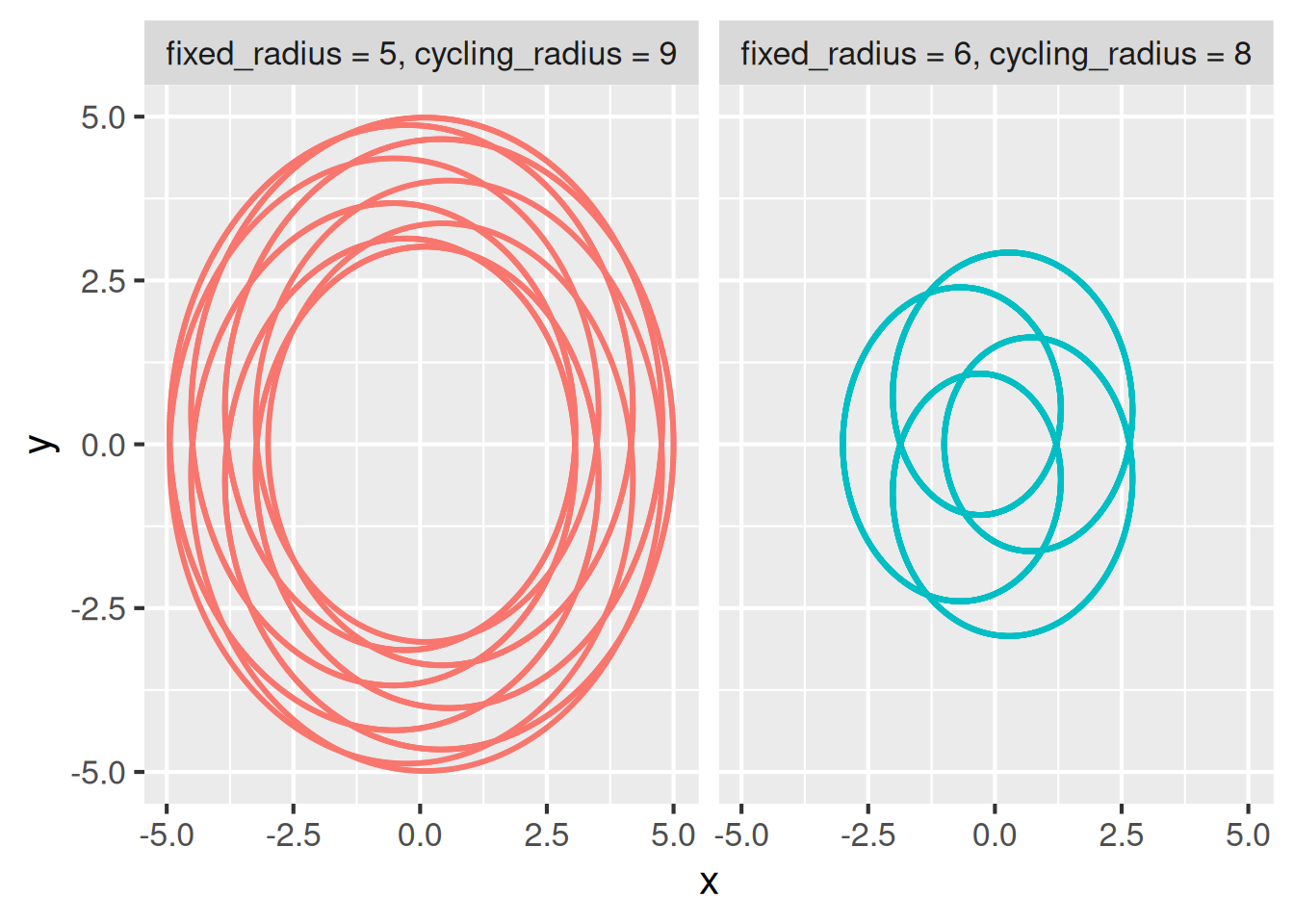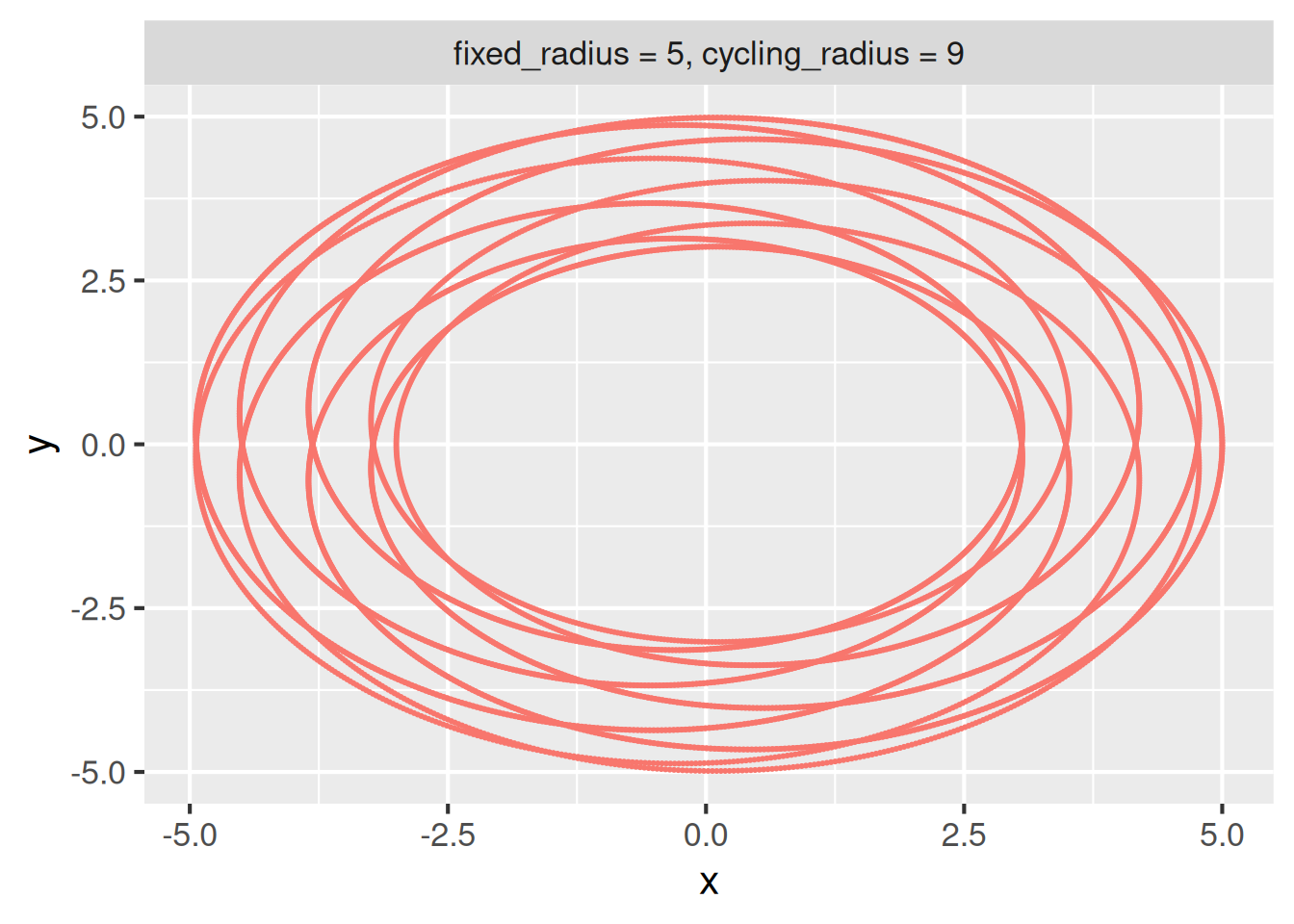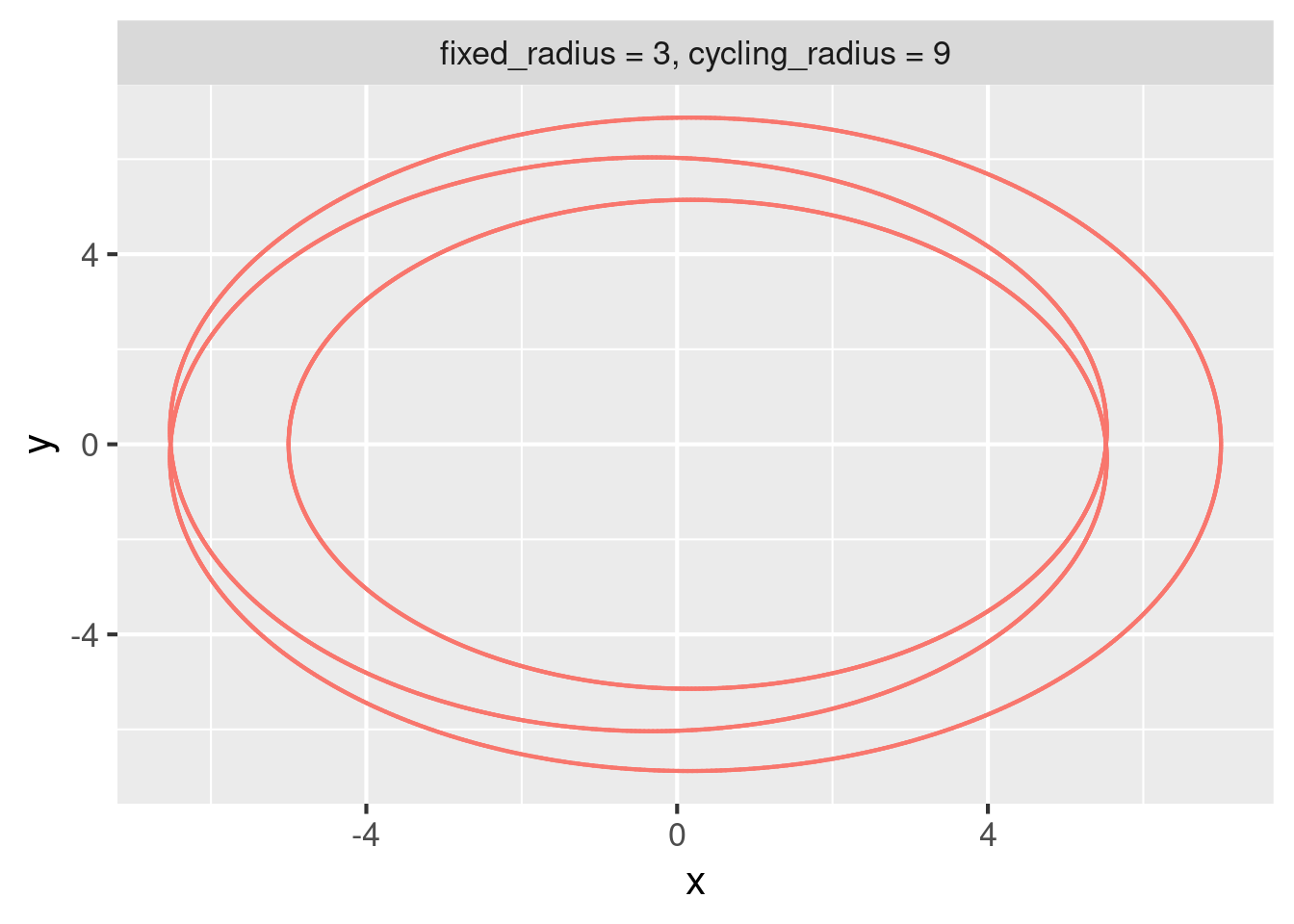# 15  Dynamic branching

Performance

Branched pipelines can be computationally demanding. See the performance chapter for options, settings, and other choices to optimize and monitor large pipelines.

## 15.1 Branching

Sometimes, a pipeline contains more targets than a user can comfortably type by hand. For projects with hundreds of targets, branching can make the code in `_targets.R` shorter and more concise.

`targets` supports two types of branching: dynamic branching and static branching. Some projects are better suited to dynamic branching, while others benefit more from static branching or a combination of both. Here is a short list of tradeoffs.

Dynamic Static
Pipeline creates new targets at runtime. All targets defined in advance.
Cryptic target names. Friendly target names.
Scales to hundreds of branches. Does not scale as easily for `tar_visnetwork()` etc.
No metaprogramming required. Familiarity with metaprogramming is helpful.

Dynamic branching is the act of defining new targets (called branches) while the pipeline is running (e.g. during `tar_make()`). Prior to launching the pipeline, the user does not need to know the number of branches or the input data of each branch.

To use dynamic branching, set the `pattern` argument of `tar_target()`. The pattern determines how dynamic branches are created and how the input data is partitioned among the branches. A branch is single iteration of the target’s command on a single piece of the input data. Branches are automatically created based on how the input data breaks into pieces, and `targets` automatically combines the output from all the branches when you reference the dynamic target as a whole.

## 15.3 Example

To illustrate, consider the example pipeline below. It uses dynamic branching to generate random spirographs using code borrowed from W. Joel Schneider’s `spiro` package.1. A spirograph is a type of two-dimensional algebraic curve determined (in part) by parameters `fixed_radius` and `cycling_radius`. Targets `fixed_radius` and `cycling_radius` draw random parameter values, and the dynamic target `points` generates a spirograph dataset for each set of parameters (one spirograph per dynamic branch). Target `single_plot` plots each spirograph separately, and `combine_plot` plots all the spirographs together.

``````# _targets.R
library(ggplot2)
library(targets)
library(tibble)

t <- seq(1, 30 * pi, length.out = 1e4)
x <- diff * cos(t) + cos(t * ratio)
y <- diff * sin(t) - sin(t * ratio)
}

plot_spirographs <- function(points) {
ggplot(points) +
geom_point(aes(x = x, y = y, color = parameters), size = 0.1) +
facet_wrap(~parameters) +
theme_gray(16) +
guides(color = "none")
}

list(
tar_target(fixed_radius, sample.int(n = 10, size = 2)),
tar_target(cycling_radius, sample.int(n = 10, size = 2)),
tar_target(
points,
),
tar_target(
single_plot,
plot_spirographs(points),
pattern = map(points),
iteration = "list"
),
tar_target(combined_plot, plot_spirographs(points))
)``````
``````tar_make()
#> ● built target cycling_radius [0.001 seconds]
#> ● built target fixed_radius [0 seconds]
#> ▶ start branch points_3755930c
#> ● built branch points_3755930c [0.004 seconds]
#> ▶ start branch points_04fd8553
#> ● built branch points_04fd8553 [0.007 seconds]
#> ● built pattern points
#> ▶ start target combined_plot
#> ● built target combined_plot [0.018 seconds]
#> ▶ start branch single_plot_7de29139
#> ● built branch single_plot_7de29139 [0.012 seconds]
#> ▶ start branch single_plot_dda5f5ba
#> ● built branch single_plot_dda5f5ba [0.006 seconds]
#> ● built pattern single_plot
#> ▶ end pipeline [0.307 seconds]``````

The final plot shows all the spirographs together.

``tar_read(combined_plot)``This plot comes from all the branches of `points` aggregated together. Because target `points` has `iteration = "vector"` in `tar_target()`, any reference to the whole target automatically aggregates the branches using `vctrs::vec_c()`. For data frames, this just binds all the rows.

``````tar_read(points)
#> # A tibble: 20,000 × 4
#>    <dbl> <dbl>        <int>          <int>
#>  1  2.40  2.39            7              3
#>  2  2.35  2.41            7              3
#>  3  2.31  2.43            7              3
#>  4  2.27  2.44            7              3
#>  5  2.22  2.46            7              3
#>  6  2.18  2.48            7              3
#>  7  2.13  2.49            7              3
#>  8  2.09  2.51            7              3
#>  9  2.04  2.53            7              3
#> 10  2.00  2.54            7              3
#> # ℹ 19,990 more rows``````

By contrast, target `single_plot` list of branches because of `iteration = "list"`.

``````tar_load(single_plot)
class(single_plot)
#>  "list"
length(single_plot)
#>  2``````

Use the `branches` argument of `tar_read()` to read an individual branch or subset of branches.

``````tar_read(single_plot, branches = 1)
#> \$single_plot_7de29139``````## 15.4 Provenance

Recall our dynamic target `points` with branches for spirograph datasets. Each branch has columns `fixed_radius` and `cycling_radius` so we know which parameter set each spirograph used. It is good practice to proactively append this metadata to each branch, e.g. in `spirograph_points()`. That way, if a branch errors out, it is easy to track down the upstream data that caused it.2

``````tar_read(points, branches = 1) # first branch
#> # A tibble: 10,000 × 4
#>    <dbl> <dbl>        <int>          <int>
#>  1  2.40  2.39            7              3
#>  2  2.35  2.41            7              3
#>  3  2.31  2.43            7              3
#>  4  2.27  2.44            7              3
#>  5  2.22  2.46            7              3
#>  6  2.18  2.48            7              3
#>  7  2.13  2.49            7              3
#>  8  2.09  2.51            7              3
#>  9  2.04  2.53            7              3
#> 10  2.00  2.54            7              3
#> # ℹ 9,990 more rows``````
``````tar_read(points, branches = 2) # second branch
#> # A tibble: 10,000 × 4
#>    <dbl> <dbl>        <int>          <int>
#>  1 0.631  2.38            4              1
#>  2 0.604  2.43            4              1
#>  3 0.577  2.47            4              1
#>  4 0.551  2.51            4              1
#>  5 0.526  2.55            4              1
#>  6 0.501  2.60            4              1
#>  7 0.478  2.64            4              1
#>  8 0.454  2.68            4              1
#>  9 0.432  2.72            4              1
#> 10 0.410  2.76            4              1
#> # ℹ 9,990 more rows``````

## 15.5 Patterns

`targets` supports many more types of dynamic branching patterns.

• `map()`: one branch per tuple of elements.
• `cross()`: one branch per combination of elements.
• `slice()`: select individual pieces to branch over. For example, `pattern = slice(x, index = c(3, 4))` branches over the third and fourth slices (or branches) of target `x`.
• `head()`: branch over the first few elements.
• `tail()`: branch over the last few elements.
• `sample()`: branch over a random subset of elements.

Patterns are composable. For example, `pattern = cross(other_parameter, map(fixed_radius, cycling_radius))` is conceptually equivalent to `tidyr::crossing(other_parameter, tidyr::nesting(fixed_radius, cycling_radius))`. You can test and experiment with branching structures using `tar_pattern()`. In the output below, suffixes `_1`, `_2`, and `_3`, denote both dynamic branches and the slices of upstream data they branch over.

``````tar_pattern(
other_parameter = 3,
)
#> # A tibble: 6 × 3
#>   <chr>             <chr>          <chr>

## 15.6 Iteration

The `iteration` argument of `tar_target()` determines how to split non-dynamic targets and how to aggregate dynamic ones. Consider the following subset of the spirograph pipeline. Below, `iteration` is equal to `"vector"` by default.

``````list(
tar_target(fixed_radius, sample.int(n = 10, size = 2)),
tar_target(cycling_radius, sample.int(n = 10, size = 2)),
tar_target(
points,
),
tar_target(combined_plot, plot_spirographs(points))
)``````

For non-dynamic targets `fixed_radius` and `cycling_radius`, `iteration = "vector"` means that downstream dynamic targets branch over slices from `vctrs::vec_slice()`. In other words, the first branch of `points` is `spirograph_points(vec_slice(fixed_radius, 1), vec_slice(cycling_radius, 1))`, and second branch of `points` is `spirograph_points(vec_slice(fixed_radius, 2), vec_slice(cycling_radius, 2))`. Since `fixed_radius` is a numeric vector, `vec_slice(fixed_radius, 1)` is a vector of length 1. (But if `fixed_radius` were a data frame, then `vec_slice(fixed_radius, 1)` would be a one-row data frame). If `iteration` were equal to `"list"` for `fixed_radius` and `cycling_radius`, then the first and second branches would instead be `spirograph_points(fixed_radius[], cycling_radius[])` and `spirograph_points(fixed_radius[])`, respectively.

For dynamic target `points`, `iteration = "vector"` means all the branches are aggregated with `vctrs::vec_c()`. Since each branch of `points` is a data frame, aggregation is equivalent to `dplyr::bind_rows()` in this case. That is why, as previously shown, `tar_read(points)` returns a monolithic data frame. `points` becomes a monolithic data frame also when non-dynamic target `combined_plot` runs its command `plot_spirographs(points)`. In other words, `plot_spirographs(points)` is equivalent to `plot_spirographs(vec_c(points_940d58fc, points_bf28fe5a))`, where `points_940d58fc` and `points_bf28fe5a` are the individual branches of `points`.3. If `iteration` were equal to `"list"` for `points`, then `plot_spirographs(points)` in `combined_plot` would be equivalent to `plot_spirographs(list(points_940d58fc, points_bf28fe5a))`.

`iteration = "group"` is for dynamic branching across `dplyr::group_by()` row groups of a data frame, and it is covered in the next section.

## 15.7 Branching over row groups

To branch over `dplyr::group_by()` row groups of a data frame, use `iteration = "group"` together with `tar_group()`. To demonstrate, consider the following alternative version of the spirograph pipeline. Below, we start with a monolithic data frame with all the spirographs together, and then we branch over the row groups of that data frame to create one visual for each dynamic branch.

``````# _targets.R
library(dplyr)
library(ggplot2)
library(targets)
library(tibble)

t <- seq(1, 30 * pi, length.out = 1e4)
x <- diff * cos(t) + cos(t * ratio)
y <- diff * sin(t) - sin(t * ratio)
}

plot_spirographs <- function(points) {
ggplot(points) +
geom_point(aes(x = x, y = y, color = parameters), size = 0.1) +
facet_wrap(~parameters) +
theme_gray(16) +
guides(color = "none")
}

list(
tar_target(
points,
bind_rows(
spirograph_points(3, 9),
spirograph_points(7, 2)
) %>%
tar_group(),
iteration = "group"
),
tar_target(
single_plot,
plot_spirographs(points),
pattern = map(points),
iteration = "list"
)
)``````
``````tar_make()
#> ▶ start target points
#> ● built target points [0.018 seconds]
#> ▶ start branch single_plot_d464a6a6
#> ● built branch single_plot_d464a6a6 [0.03 seconds]
#> ▶ start branch single_plot_4d5f6e9c
#> ● built branch single_plot_4d5f6e9c [0.021 seconds]
#> ● built pattern single_plot
#> ▶ end pipeline [0.253 seconds]``````
``````tar_read(single_plot, branches = 1)
#> \$single_plot_d464a6a6``````The `tar_group_by()` function in `tarchetypes` makes this branching easier. Using `tar_group_by()`, the pipeline condenses down to:

``````list(
tar_group_by(
points,
bind_rows(
spirograph_points(3, 9),
spirograph_points(7, 2)
),
),
tar_target(
single_plot,
plot_spirographs(points),
pattern = map(points),
iteration = "list"
)
)``````

For similar functions that branch across row groups, visit https://docs.ropensci.org/tarchetypes/reference/index.html#dynamic-grouped-data-frames.

## 15.8 Branching over files

Dynamic branching over files is tricky. A target with `format = "file"` treats the entire set of files as an irreducible bundle. That means in order to branch over files downstream, each file must already have its own branch. Here is a pipeline that begins with spirograph data files and loads each into a different dynamic branch.

``````# _targets.R
library(targets)
list(
tar_target(paths, c("spirograph_dataset_1.csv", "spirograph_dataset_1.csv")),
tar_target(files, paths, format = "file", pattern = map(paths)),
)``````

The `tar_files()` function from the `tarchetypes` package is shorthand for the first two targets above.

``````# _targets.R
library(targets)
library(tarchetypes)
list(
tar_files(files, c("spirograph_dataset_1.csv", "spirograph_dataset_1.csv")),
)``````

## 15.9 Performance and batching

Dynamic branching makes it easy to create many targets. Unfortunately, if the number of targets exceeds several thousand, overhead may build up and the package may slow down. Temporary workarounds can avoid overhead in specific cases: for example, the `shortcut` argument of `tar_make()`, and choosing a pattern like `slice()` or `head()` instead of a full `map()`. But to minimize overhead at scale, it is better to accomplish the same amount of work with a fewer number of targets. In other words, do more work inside each dynamic branch.

Batching is particularly useful to reduce overhead. In batching, each dynamic branch performs multiple computations instead of just one. The `tarchetypes` package supports several general-purpose functions that do batching automatically: most notably `tar_rep()` and `tar_map_rep()` for simulation studies and `tar_group_count()`, `tar_group_size()`, and `tar_group_select()` for batching over the rows of a data frame.

The packages in the R Targetopia support batching for specific use cases. For example, in `stantargets`, `tar_stan_mcmc_rep_summary()` dynamically branches over batches of simulated datasets for Stan models.

The `targets-stan` repository has an example of custom batching implemented from scratch. The goal of the pipeline is to validate a Bayesian model by simulating thousands of dataset, analyzing each with a Bayesian model, and assessing the overall accuracy of the inference. Rather than define a target for each dataset in model, the pipeline breaks up the work into batches, where each batch has multiple datasets or multiple analyses. Here is a version of the pipeline with 40 batches and 25 simulation reps per batch (1000 reps total in a pipeline of 82 targets).

``````# _targets.R
library(targets)
list(
tar_target(model_file, compile_model("stan/model.stan"), format = "file"),
tar_target(index_batch, seq_len(40)),
tar_target(index_sim, seq_len(25)),
tar_target(
data_continuous,
purrr::map_dfr(index_sim, ~simulate_data_continuous()),
pattern = map(index_batch)
),
tar_target(
fit_continuous,
map_sims(data_continuous, model_file = model_file),
pattern = map(data_continuous)
)
)``````

1. The pipeline uses code borrowed from the `spiro` package. The code in the `spirograph_points()` function is adapted from https://github.com/wjschne/spiro/blob/87f73ec37ceb0a7a9d09856ada8ae28d587a2ebd/R/spirograph.R under the CC0 1.0 Universal license: https://github.com/wjschne/spiro/blob/87f73ec37ceb0a7a9d09856ada8ae28d587a2ebd/LICENSE.md↩︎

2. `points_940d58fc` and `points_bf28fe5a` are targets in their own right and can be inspected with `tar_read(points_940d58fc)` and `tar_read(points_bf28fe5a)`↩︎